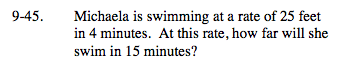Home > CC2MN > Chapter mc29 > Lesson mc29.2.1 > Problem9-45

9-45.

Michaela is swimming at a rate of 25 feet in 4 minutes. At this rate, how far will she swim in 15 minutes? Homework Help ✎Identify this as a proportional relationship problem.

You know that 25 feet is to 4 minutes.
Now use one of the three methods of solving proportions to find the answer to this problem.

$\text{Cross multiplication method: }\frac{\text{25 feet}}{\text{4 minutes}} = \frac{x\text{ feet}}{\text{15 minutes}}$

25(15) = x(4) Solve for x.

93.75 feet